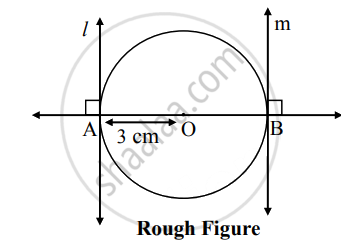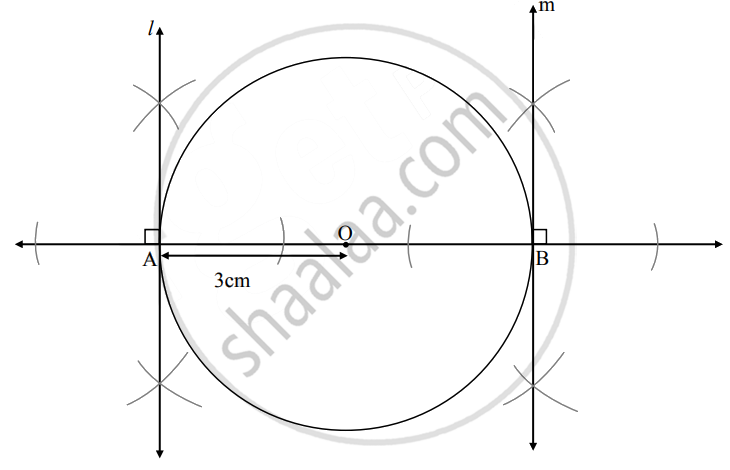# Draw a circle with a diameter AB of length 6 cm. Draw a tangent to the circle from the end points of the diameter. - Geometry

Diagram

Draw a circle with a diameter AB of length 6 cm. Draw a tangent to the circle from the end points of the diameter.

#### Solution

Analysis:

Diameter = 6 cm

{:("seg OA" ⊥ "line"  l),("seg OB" ⊥ "line m"):}}  ......[Tangent is perpendicular to radius]

The perpendicular to seg OA and seg OB at points A and B respectively will give the required tangents at A and B.Steps of construction:

1. With center O, draw a circle of radius 3 cm.
2. Draw a chord AB of length 6 cm.
3. Draw rays OA and OB.
4. Draw line l ⊥ ray OA at point A.
5. Draw line m ⊥ ray OB at point B.
Lines l and m are the required tangents to the circle.
Concept: Construction of a Tangent to the Circle at a Point on the Circle
Is there an error in this question or solution?

Share